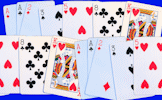# Compound Interest Calculator

## A customised online calculator for quickly finding the solutions to compound interest problems.

##### Compound Interest CalculatorOnline ExerciseExam-StyleMore Calculator Activities
Present Value (PV): \$ £ € ¥ ₹ 元 THB %

Compound interest can be calculated by using the following formula to calculate the final amount or future value (FV)

$$FV = PV(1 + \frac{r}{100})^n$$

$$PV$$ is the present value.

$$n$$ is the number of years.

$$r$$ is the rate of interest.

or by using the iterative function

$$x_{n+1} = x_n + \frac{x_n \times r}{100}$$

where $$x_0$$ is the present value [See Calculator Workout Skill 16]

$$FV = PV(1 + \frac{r}{100k})^{kn}$$

$$PV$$ is the present value.

$$n$$ is the number of years.

$$r$$ is the rate of interest.

$$k$$ is the number of compounding periods per year.

To calculate depreciation use a negative interest rate (r).

## Transum.org

This web site contains over a thousand free mathematical activities for teachers and pupils. Click here to go to the main page which links to all of the resources available.## More Activities:

Mathematicians are not the people who find Maths easy; they are the people who enjoy how mystifying, puzzling and hard it is. Are you a mathematician?

Comment recorded on the 21 October 'Starter of the Day' page by Mr Trainor And His P7 Class(All Girls), Mercy Primary School, Belfast:

"My Primary 7 class in Mercy Primary school, Belfast, look forward to your mental maths starters every morning. The variety of material is interesting and exciting and always engages the teacher and pupils. Keep them coming please."

Comment recorded on the 9 May 'Starter of the Day' page by Liz, Kuwait:

"I would like to thank you for the excellent resources which I used every day. My students would often turn up early to tackle the starter of the day as there were stamps for the first 5 finishers. We also had a lot of fun with the fun maths. All in all your resources provoked discussion and the students had a lot of fun."

Each month a newsletter is published containing details of the new additions to the Transum website and a new puzzle of the month.

The newsletter is then duplicated as a podcast which is available on the major delivery networks. You can listen to the podcast while you are commuting, exercising or relaxing.

Transum breaking news is available on Twitter @Transum and if that's not enough there is also a Transum Facebook page.

#### Playing Card MathsImagine you are on a desert island with nothing but a pack of playing cards. Do you have to stop learning mathematics? Of course not! Here are some great ideas for teachers, parents and tutors.

## Numeracy

"Numeracy is a proficiency which is developed mainly in Mathematics but also in other subjects. It is more than an ability to do basic arithmetic. It involves developing confidence and competence with numbers and measures. It requires understanding of the number system, a repertoire of mathematical techniques, and an inclination and ability to solve quantitative or spatial problems in a range of contexts. Numeracy also demands understanding of the ways in which data are gathered by counting and measuring, and presented in graphs, diagrams, charts and tables."

Secondary National Strategy, Mathematics at key stage 3

## Go Maths

Learning and understanding Mathematics, at every level, requires learner engagement. Mathematics is not a spectator sport. Sometimes traditional teaching fails to actively involve students. One way to address the problem is through the use of interactive activities and this web site provides many of those. The Go Maths main page links to more activities designed for students in upper Secondary/High school.

## Teachers

If you found this activity useful don't forget to record it in your scheme of work or learning management system. The short URL, ready to be copied and pasted, is as follows:

Alternatively, if you use Google Classroom, all you have to do is click on the green icon below in order to add this activity to one of your classes.

It may be worth remembering that if Transum.org should go offline for whatever reason, there are mirror sites at Transum.com and Transum.info that contain most of the resources that are available here on Transum.org.

When planning to use technology in your lesson always have a plan B!

Do you have any comments? It is always useful to receive feedback and helps make this free resource even more useful for those learning Mathematics anywhere in the world. Click here to enter your comments.For All: